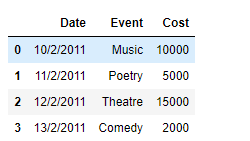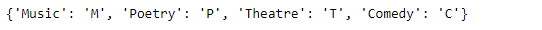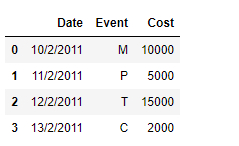# Using dictionary to remap values in Pandas DataFrame columns

While working with data in Pandas, we perform a vast array of operations on the data to get the data in the desired form. One of these operations could be that we want to remap the values of a specific column in the DataFrame. Let’s discuss several ways in which we can do that.

Given a Dataframe containing data about an event, remap the values of a specific column to a new value.

Code #1: We can use `DataFrame.replace()` function to achieve this task. Let’s see how we can do that.

 `# importing pandas as pd ` `import` `pandas as pd ` ` `  `# Creating the DataFrame ` `df ``=` `pd.DataFrame({``'Date'``:[``'10/2/2011'``, ``'11/2/2011'``, ``'12/2/2011'``, ``'13/2/2011'``], ` `                    ``'Event'``:[``'Music'``, ``'Poetry'``, ``'Theatre'``, ``'Comedy'``], ` `                    ``'Cost'``:[``10000``, ``5000``, ``15000``, ``2000``]}) ` ` `  `# Print the dataframe ` `print``(df) `

Output :Now we will remap the values of the ‘Event’ column by their respective codes.

 `# Create a dictionary using which we ` `# will remap the values ` `dict` `=` `{``'Music'` `: ``'M'``, ``'Poetry'` `: ``'P'``, ``'Theatre'` `: ``'T'``, ``'Comedy'` `: ``'C'``} ` ` `  `# Print the dictionary ` `print``(``dict``) ` ` `  `# Remap the values of the dataframe ` `df.replace({``"Event"``: ``dict``}) `

Output :Code #2: We can use `map()` function to achieve this task.

 `# importing pandas as pd ` `import` `pandas as pd ` ` `  `# Creating the DataFrame ` `df ``=` `pd.DataFrame({``'Date'``:[``'10/2/2011'``, ``'11/2/2011'``, ``'12/2/2011'``, ``'13/2/2011'``], ` `                    ``'Event'``:[``'Music'``, ``'Poetry'``, ``'Theatre'``, ``'Comedy'``], ` `                    ``'Cost'``:[``10000``, ``5000``, ``15000``, ``2000``]}) ` ` `  `# Print the dataframe ` `print``(df) `

Output :Now we will remap the values of the ‘Event’ column by their respective codes.

 `# Create a dictionary using which we ` `# will remap the values ` `dict` `=` `{``'Music'` `: ``'M'``, ``'Poetry'` `: ``'P'``, ``'Theatre'` `: ``'T'``, ``'Comedy'` `: ``'C'``} ` ` `  `# Print the dictionary ` `print``(``dict``) ` ` `  `# Remap the values of the dataframe ` `df[``'Event'``]``=` `df[``'Event'``].``map``(``dict``) ` ` `  `# Print the DataFrame after modification ` `print``(df) `

Output :My Personal Notes arrow_drop_upCheck out this Author's contributed articles.

If you like GeeksforGeeks and would like to contribute, you can also write an article using contribute.geeksforgeeks.org or mail your article to contribute@geeksforgeeks.org. See your article appearing on the GeeksforGeeks main page and help other Geeks.

Please Improve this article if you find anything incorrect by clicking on the "Improve Article" button below.

Article Tags :

2

Please write to us at contribute@geeksforgeeks.org to report any issue with the above content.